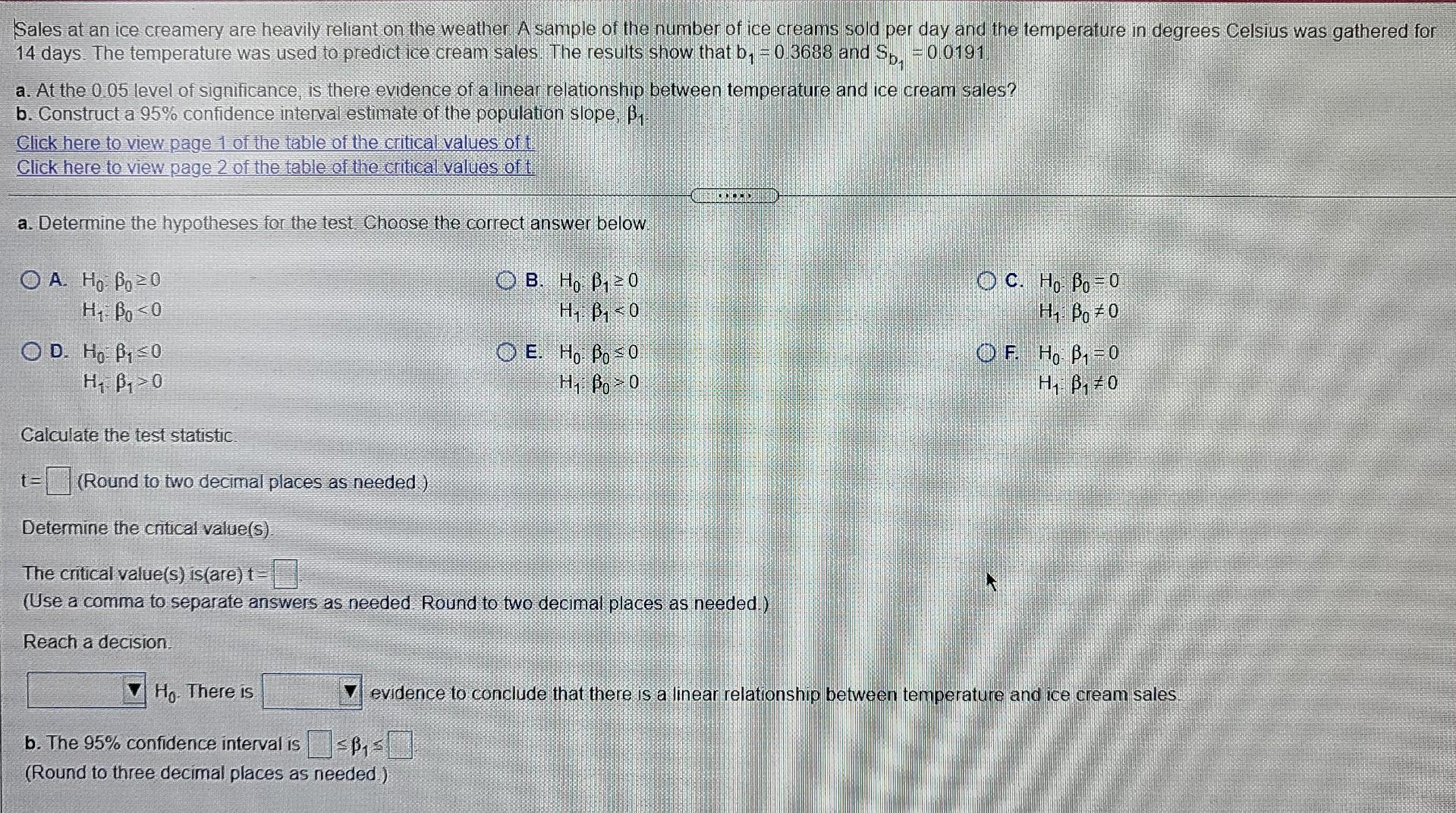# Question Sales at an ice creamery are heavily reliant on the weather A sample of the number of ice creams sold per day and the temperature in degrees Celsius was gathered for 14 days. The temperature was used to predict ice cream sales. The results show that b150.3688 and Sb, 00191 a. At the 0 05 level of significance, is there evidence of a linear relationship between temperature and ice cream sales? b. Construct a 95% confidence interval estimate of the population slope, Bi. Click here to view page 1 of the table of the critical values of t Click here to view page 2 of the table of the critical values of t. COLOR a. Determine the hypotheses for the test Choose the correct answer below. OA Ho Bo=0 H7 Bo<0 OD. Ho: B, 0 H, B,> 0 O B. Ho B120 H-: , <0 O E. Ho Bo = 0 Hi Bo > 0 O C. Ho Bo=0 H1: Bo 70 O F. Ho B1 = 0 H, B,+ 0 Calculate the test statistic t=(Round to two decimal places as needed ) Determine the cntical value(s). The critical value(s) is(are) t= (Use a comma to separate answers as needed Round to two decimal places as needed.) Reach a decision. Ho There is evidence to conclude that there is a linear relationship between temperature and ice cream sales b. The 95% confidence interval is <B> (Round to three decimal places as needed.)6XM0X4 The Asker · Probability and StatisticsTranscribed Image Text: Sales at an ice creamery are heavily reliant on the weather A sample of the number of ice creams sold per day and the temperature in degrees Celsius was gathered for 14 days. The temperature was used to predict ice cream sales. The results show that b150.3688 and Sb, 00191 a. At the 0 05 level of significance, is there evidence of a linear relationship between temperature and ice cream sales? b. Construct a 95% confidence interval estimate of the population slope, Bi. Click here to view page 1 of the table of the critical values of t Click here to view page 2 of the table of the critical values of t. COLOR a. Determine the hypotheses for the test Choose the correct answer below. OA Ho Bo=0 H7 Bo<0 OD. Ho: B, 0 H, B,> 0 O B. Ho B120 H-: , <0 O E. Ho Bo = 0 Hi Bo > 0 O C. Ho Bo=0 H1: Bo 70 O F. Ho B1 = 0 H, B,+ 0 Calculate the test statistic t=(Round to two decimal places as needed ) Determine the cntical value(s). The critical value(s) is(are) t= (Use a comma to separate answers as needed Round to two decimal places as needed.) Reach a decision. Ho There is evidence to conclude that there is a linear relationship between temperature and ice cream sales b. The 95% confidence interval is (Round to three decimal places as needed.)
More
Transcribed Image Text: Sales at an ice creamery are heavily reliant on the weather A sample of the number of ice creams sold per day and the temperature in degrees Celsius was gathered for 14 days. The temperature was used to predict ice cream sales. The results show that b150.3688 and Sb, 00191 a. At the 0 05 level of significance, is there evidence of a linear relationship between temperature and ice cream sales? b. Construct a 95% confidence interval estimate of the population slope, Bi. Click here to view page 1 of the table of the critical values of t Click here to view page 2 of the table of the critical values of t. COLOR a. Determine the hypotheses for the test Choose the correct answer below. OA Ho Bo=0 H7 Bo<0 OD. Ho: B, 0 H, B,> 0 O B. Ho B120 H-: , <0 O E. Ho Bo = 0 Hi Bo > 0 O C. Ho Bo=0 H1: Bo 70 O F. Ho B1 = 0 H, B,+ 0 Calculate the test statistic t=(Round to two decimal places as needed ) Determine the cntical value(s). The critical value(s) is(are) t= (Use a comma to separate answers as needed Round to two decimal places as needed.) Reach a decision. Ho There is evidence to conclude that there is a linear relationship between temperature and ice cream sales b. The 95% confidence interval is (Round to three decimal places as needed.)